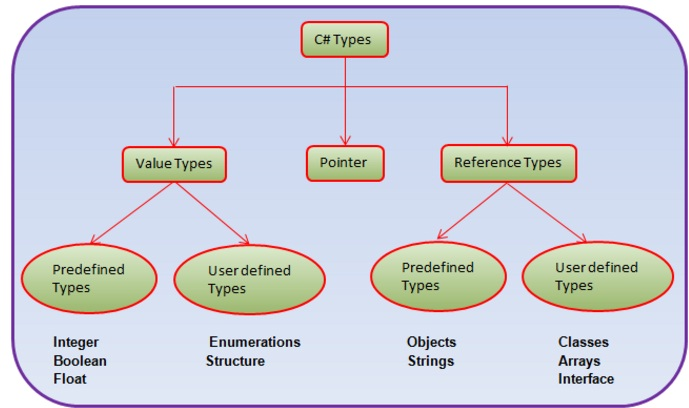# C# Data Types

There are two kinds of data types in C#:
• Value
• Reference
If you declared a variable using any basic, built-in data types or a user defined structure, it will be a value type.

Exception: Always remember, the string data type, is a reference type not value type.
A value type contents are stored on the stack.

For example:
int intVar=50;
The value 50 is stored in an area of memory called the stack.
You will use the intVar variable in the function, when the control goes out of scope of the method, the value from the stack will be over.

Using the stack is efficient compare to heap, but it is problem if control goes out of scope. If you created reference type such as an instance of a class, then you can share data between different classes.
For more understanding of value types and reference types let us take example.

#### Example 1

int  x = new int();
x = 120;
int  y = new int();
y = x;
y = 130;
Console.WriteLine("x = " +x);

Output: x= 120

In the above example, value of variable y is changed. "x" has its original value and its  location on stack is not affected . Therefore output is x= 120.

#### Example 2

class Numbers
{
public int  intVar;
}
class Program
{
static void Main(string[] args)
{
Numbers obj1 = new Numbers();
obj1.intVar = 30;
Numbers obj2 = new Numbers();
obj2 = obj1;
obj2.intVar = 40;
Console.WriteLine("Value = "+obj1.intVar);
}
}

Output: Value = 40

In the above program, both variable x and y are pointing to the same location on the heap memory.## Difference between Value types and Reference types.

Value TypeReference Type
Stored on stackStored on heap
Contains actual valueContains reference to a value
Cannot contain null valuesCan contain null values
Value type is erased on its own from stack when control goes out of scope.It requires garbage collector to free memory.
Memory is allocated at compile timeMemory is allocated at run time

## Boxing and Unboxing

Boxing and unboxing is an important concept in .NET framework. Boxing and unboxing enables you to convert value type into reference type and vice versa.

Boxing: Converting a value type to reference type is called as Boxing. It is implicit conversion.

#### Example

int intVar = 10;                                  // intVar is a value type
object obj = intVar;                            // intVar is boxed
System.Console.WriteLine(intVar.ToString());

Unboxing: Converting a reference type into value type is called unboxing. It is an explicit operation.

#### Example

using System.Collections;
ArrayList list  =  new ArrayList();   // list is a reference type
int intVar = 10;                               // intVar is a value type
intVar = (int)list;                        // list is unboxed
System.Console.WriteLine(intVar.ToString());

## Nullable Types

nullable types is a special types in C#, in which you can assign normal values as well as null values. nullable types comes under the value types. A nullable type has the same range and characteristics as its fundamental or built in type.

Nullable types are instances of the System.Nullable<T> struct>, where T must be a non nullable value type.

You can create nullable types in two different ways. First, you can explicitly declare
objects of type Nullable<T.

Example:
System.Nullable<int>  intVar;
System.Nullable<bool> flag;

It will creates int and bool nullable types.

Second way, which is also sweet and short, is given below. You can create a value type as nullable by using the ? symbol after the value type.

int ? count;
bool ? done;
int ? x = null.

There are many scenarios in real life where nullable types are very important. Suppose that there is a column in database table of type integer and the value in database is null. If we want to use this database value or assign to other variable in C#, previously there was no way but now we can use nullable types.

#### Properties

HasValue: It indicates whether variable has value of its underlying type. If it has value then it returns true otherwise false.
Value: Gets the value of the current nullable type variable.

#### Methods

GetValueOrDefault(): Retrieves the value of the current Nullable<T> object, or the object's default value.

HasValue property returns true if the nullable type variable has been assigned a value; otherwise it will return false.

Accessing the value using intVar.Value (NullableType.value) will throw a runtime exception if we assign null or not assigned any value.

static void Main(string[ ] args)
{
int ? intVar = null;
Console.WriteLine("intVar has  value: " + intVar.Value);
}

The above code will give runtime exception.

#### Example

using System;
namespace ConsoleApplication1
{
class Program
{
static void Main(string[ ] args)
{
int? intVar = null;
if (intVar.HasValue)
Console.WriteLine("intVar has  value: " + intVar.Value);
else
{
Console.WriteLine("intVar has no value");
Console.WriteLine("intVar = "+intVar.GetValueOrDefault());
}
intVar = 35;
if (intVar.HasValue)
Console.WriteLine("intVar has  value: " + intVar.Value);
else
Console.WriteLine("intVar has no value");
}
}
}

Output:
intVar has no value
intVar =0
intVar has value = 35

NOTE: Nested nullable types are not allowed. Nullable<Nullable<int>> intVar. It will give a compile time error.

## Static Nullable helper class

Comparison operators do not work against null. Let us take example.

#### Example

using System;
namespace ConsoleApplication1
{
class Program
{
static void Main(string[] args)
{
int? firstVar = null;
int secondVar = 100;
if (firstVar < secondVar)
Console.WriteLine("intVar < secondVar");
else if (firstVar > 100)
Console.WriteLine("intVar > secondVar");
else if (firstVar == 100)
Console.WriteLine("intVar == secondVar");
else
Console.WriteLine("Could not compare null");
}
}
}

Output:
Could not compare null

Nullable static class has a compare() method to compare nullable types.

#### Example

using System;
namespace ConsoleApplication1
{
class Program
{
static void Main(string[] args)
{
int? firstVar = null;
int secondVar = 100;
if (Nullable.Compare<int>(firstVar, secondVar) < 0)
Console.WriteLine("firstVar < secondVar");
else if (Nullable.Compare<int>(firstVar, secondVar) > 0)
Console.WriteLine("firstVar > secondVar");
else
Console.WriteLine("firstVar = secondVar");